Definitions of Square Dance Calls and Concepts
Cross Flip The Line ({fraction}) [C3B]

Index -->  Plus  |  A1  |  A2  |  C1  |  C2  |  C3A  |  C3B  |  C4  |  NOL  |
Definitions (Text Only) -->  Plus  |  A1  |  A2  |  C1  |  C2  |  C3A  |  C3B  |  C4  |  NOL  |
 Find call:

 \$B8@8l(BTwo-Faced Line \$B\$+\$i(B.

1 \$B\$D\$NF0\$-\$H\$7\$F(B, Centers Cross Run \$B\$r\$7\$FA40w\$G(B Any Shoulder Tag The Line \$B\$rM?\$(\$i\$l\$?(B fractional \$B\$N(B Tag formation \$B\$^\$G9T\$\$\$^\$9(B. Fraction \$B\$,\$J\$\$\$H\$-\$O(B, full Tag The Line \$B\$N0LCV\$^\$G9T\$-\$^\$9(B.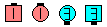\$B\$B;O\$a\$N(B formation: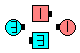1/4 Cross Flip The Line\$B\$N8e(B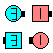1/2 Cross Flip The Line\$B\$N8e(B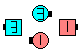3/4 Cross Flip The Line\$B\$N8e(B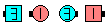Cross Flip The Line\$B\$N8e(B

\$BCm
• Tag The Line \$B\$HF1\$8\$h\$&\$K(B, \$B%3!<%k\$N8e\$KJ}8~\$,;X<(\$5\$l\$?\$H\$-\$O(B (\$BNc\$(\$P(B, Cross Flip The Line Left), \$B%3!<%k\$,=*\$o\$C\$?\$H\$-\$K;X<(\$5\$l\$?J}\$X(B 1/4 \$B8~\$-\$rJQ\$(\$^\$9(B.
• 1/2 Cross Flip The Line \$B\$O(B 2/3 Cross Cycle \$B\$HF1\$8\$G\$9\$,(B, 1/2 Cross Flip The Line \$B\$G\$OC/\$b(B Roll \$B\$G\$-\$^\$;\$s(B.
• \$BTagging Calls.

Cross Flip Back [C3B] (Lee Kopman 1973):
1/2 Cross Flip The Line; Scoot Back.

Cross Flip Your Neighbor [C3B] (Foggy Thompson 1973):

Cross Flip Chain Thru [C3B] (Vince Dicaudo 1973):
1/2 Cross Flip The Line; Scoot Chain Thru.

Cross Flip To A Diamond [C4] (Dick Han 1977):
1/2 Cross Flip The Line; 1/2 Box Circulate.

Cross Flip & Scatter [C3B]:
1/2 Cross Flip The Line; Scatter Scoot.

Flip The Line [C1] (Jack Lasry 1973): Wave \$B\$+\$i(B. 1 \$B\$D\$NF0\$-\$H\$7\$F(B, Centers Run \$B\$r\$7\$FA40w\$G(B Any Shoulder Tag The Line \$B\$rM?\$(\$i\$l\$?(B fractional \$B\$N(B Tag formation \$B\$^\$G9T\$\$\$^\$9(B. Fraction \$B\$,\$J\$\$\$H\$-\$O(B, full Tag The Line \$B\$N0LCV\$^\$G9T\$-\$^\$9(B.Choreography for Cross Flip The Line (fraction)Comments? Questions? Suggestions?

https://www.ceder.net/def/crossfliptheline.php?language=japan
14-December-2019 13:40:36